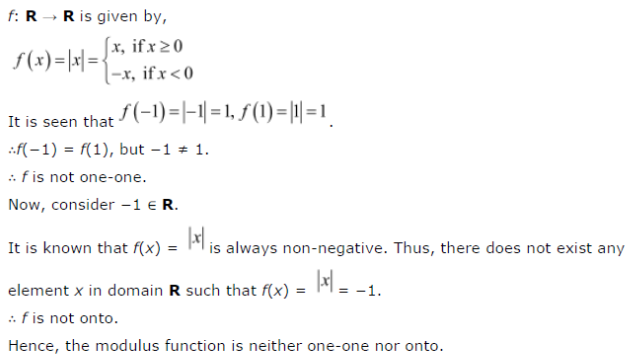# Show that the Modulus Function f : R → R, given by f(x) = |x|, is neither oneone nor onto

Show that the Modulus Function f : R → R, given by f(x) = |x|, is neither oneone nor onto, where | x | is x, if x is positive or 0 and |x| is – x, if x is negative.